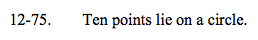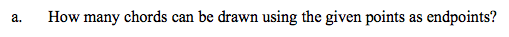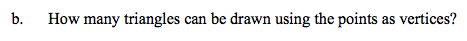### Home > A2C > Chapter 12 > Lesson 12.1.4 > Problem12-75

12-75.
1. Ten points lie on a circle. Homework Help ✎

1. How many chords can be drawn using the given points as endpoints?

2. How many triangles can be drawn using the points as vertices?10C2 = 45Use the same method as in part (a).